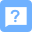2015-07-03 06:36

# 如何使用php计算图像的2D DCT？

I'm trying to find 2D DCT(The Discrete Cosine Transform) of an image using php. I have used the following equation to find it.Here is the code I have used to find the DCT.

``````function getGray(\$img,\$x,\$y){
\$col = imagecolorsforindex(\$img, imagecolorat(\$img,\$x,\$y));
return intval(\$col['red']*0.3 + \$col['green']*0.59 + \$col['blue']*0.11);
}

function  initCoefficients() {
for (\$i=1;\$i<\$this->size;\$i++)
{
\$c[\$i]=1;
}
\$c=1/sqrt(2.0);
return \$c;
}

function applyDCT(\$imagePath) {
\$color=array();

for(\$i=0;\$i<32;\$i++)
{
for(\$j=0;\$j<32;\$j++)
{
\$color[]=\$this->getGray(\$img,\$i,\$j);
}
}

\$N=32;

\$c=\$this->initCoefficients();
\$sum=0;
for (\$u=0;\$u<\$N;\$u++) {
for (\$v=0;\$v<\$N;\$v++) {

for (\$i=0;\$i<\$N;\$i++) {
for (\$j=0;\$j<\$N;\$j++) {
\$sum += (\$c[\$i]*\$c[\$j])*cos(((2*\$i+1)*\$u*pi()/(2.0*\$N)))*cos(((2*\$j+1)*\$v*pi()/(2.0*\$N)))*(\$color[\$i][\$j]);
}
}

\$sum *=sqrt(2/\$N)*sqrt(2/\$N);
\$F[\$u][\$v] = \$sum;
}
}
return \$F;
}
``````

The image size is `32*32`. My problem is, once I call the `applyDCT()`function it gives an array which has all the element values as 0.

eg:-

Array (  => Array (... ,3 => 0  => 0  => 0,...

I think problem is in my calculation. What I am doing wrong? Please help me Thank you.

#### 图片转代码服务由CSDN问答提供 功能建议``` ``` function getGray（\$ img，\$ x，\$ y）{
\$ col = imagecolorsforindex（\$ img，imagecolorat（\$ img，\$ x，\$ y））;

}

for（\$ i  = 1; \$ i＆lt; \$ this-＆gt; size; \$ i ++）
{
\$ c [\$ i] = 1;
}
\$ c  = 1 / sqrt（2.0）;
return  \$ c;
}

\$ img = \$ this-＆gt; readImageTo（\$ imagePath，32,32）;
\$ color = array（）;

for  （\$ i = 0; \$ i＆lt; 32; \$ i ++）
{
for（\$ j = 0; \$ j＆lt; 32; \$ j ++）
{
\$ color [] = \$ this-＆gt; getGray  （\$ img，\$ i，\$ j）;
}
}

\$ N = 32;

\$ n c =  \$ this-＆gt; initCoefficients（）;
\$ sum = 0;
for（\$ u = 0; \$ u＆lt; \$ N; \$ u ++）{
for（\$ v = 0; \$ v＆lt; \$ N;  \$ v ++）{

for（\$ i = 0; \$ i＆lt; \$ N; \$ i ++）{
for（\$ j = 0; \$ j＆lt; \$ N; \$ j ++）{
\$ sum + =  （\$ C [\$ i]于* \$ C [\$ J]）* COS（（（2 * \$ I + 1）* \$ U * PI（）/（2.0 * \$ N）））* COS（（（2 *  \$ j + 1）* \$ v * pi（）/（2.0 * \$ N）））*（\$ color [\$ i] [\$ j]）;
}
}

\$ sum * = sqrt  （2 / \$ N）* sqrt（2 / \$ N）;
\$ F [\$ u] [\$ v] = \$ sum;
}
}

}

图像大小为 32 * 32 。 我的问题是，一旦我调用 applyDCT（）函数，它就会给出一个数组，其中所有元素值都为0.

例如： -

数组（ =＆gt;数组（...， 3  =＆gt; 0  =＆gt; 0  =＆gt; 0，...

我认为 问题出在我的计算中。我做错了什么？请帮帮我

``````
` `
``` 写回答 好问题 提建议 追加酬金 关注问题 分享 邀请回答 ```
``` 1条回答 默认 最新 报告相同问题？ 提交 关注问题 相关推荐 更多相似问题如何使用php计算图像的2D DCT？ php 2015-07-03 06:36 回答 1 已采纳 Here is the answer baseed on the comments: \$color is not good. You're initializing it in a 1D butpython对图像dct变换后保存图像和展示图像不同的问题 python 2021-02-01 19:07 回答 4 已采纳 for i in range(len(fimg)): for j in range(len(fimg[i])): gray = fimg[i][j]*16 iDCT离散余弦变换不一致 2018-09-11 06:53 回答 1 已采纳 https://blog.csdn.net/sunshihua12829/article/details/49847625利用电脑玩android版"天天连萌"刷高分 二,利用计算机玩Android版“天天连萌”刷高分（二）——图像识别... 2021-06-19 07:14 大妈手别抖的博客 利用电脑玩Android版“天天连萌”刷高分(二)——图像识别上一篇只是提到了在PC端利用android sdk里面的工具进行截图，接下来这一篇将补充一点关于上一篇的内容，然后介绍一下程序的整个结构，以及如何进行《天天连萌...phash算法java实现_Java进阶(五十七)-基于感知哈希算法的pHash图像配准算法 2021-03-01 07:41 吹亚吹的博客 Java进阶(五十七)-基于感知哈希算法的pHash图像配准算法毕业论文提交之后，老师交给自己一项任务：图像配准，也就是给你两幅图像，通过系统来判定两幅图像是否为同一副图像。自己作为这一方面的小白，先去网上搜索... 没有解决我的问题, 去提问```
``` 点击登录 提问题 ◇ 欢迎建议意见◇ 用户帮助中心◇ 助力高考，答题得现金赢周边◇ 回答首次被采纳有奖◇ 奖惩公告 悬赏问题 ¥500 定制版本的移远EC20模块，有技术可以复制定制功能到平时不是定制版本的EC20模块里面吗 ¥450 python CV 图形面积计算 ¥200 Android APP打包后程序运行报错，求解，/AndroidRuntime: FATAL EXCEPTION: main。 ¥150 asp如何写支付宝当面付 ¥100 用Selenium-wire怎么添加请求头信息 ¥100 linux段错误问题 ¥100 okcupid相关的注册和匹配的问题 ¥100 zotero笔记内容宽度的设置 ¥100 使用pywinauto对电脑微信小程序上的问卷进行自动化填写时，填空题内容无法传入。 ¥100 测试用例排序有偿求帮助 ```
``` ```
``` window.csdn.sideToolbar = { options: { afterFinished: function () { setTimeout(function () { var helphtml = '<a class="option-box" data-type="help" href="https://ask.csdn.net/questions/7441224" target="_blank">'+'<img src="https://g.csdnimg.cn/side-toolbar/2.5/images/bangzhucopy.png" alt="" srcset="">'+ '<span class="show-txt">帮助</span></a>'; \$('.csdn-side-toolbar > a:last').before(helphtml); }, 300); }, contentEl: document.getElementsByClassName('floor') } } ```